1
In cart:
1 item\$7.99
Search and Filter Items
Search Products
OR Browse Departments

Filter Results

# Hot Dogs & Sausage

Product Size Price Quantity

CFGFL
16 oz
\$6.49

CFGFL
16 oz
\$7.99

## Bilinski Apple Chardonnay Chicken Sausage

GF
12 oz
\$6.99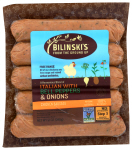## Bilinski Italian Pepper and Onion Chicken Sausage

GF
12 oz
\$6.99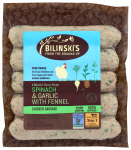## Bilinski Spinach, Garlic and Fennel Chicken Sausage

GF
12 oz
\$6.99GFOG
12 oz
\$8.99

CFL
Per Pound
\$11.99
lb

## Eichten’s Bison Sticks

CFL
Each (price approx.)
\$8.58You are ordering units, but this item is sold by the pound. The price per pound is \$25.99 and the average weight for one item is 0.33 lbs., therefore, the approximate price for one item is \$8.58. Please order the number of items you would like to receive. Your final price will be calculated based on the total weight of the actual items fulfilled.

## Eichten’s Bison Summer Sausage

CFL
Per Pound
\$17.99
lbCFL
16 oz
\$9.99

CFL
12 oz
\$9.99

## Gerhard’s Original Brats

CFL
12 oz
\$7.99 1 in cart Go to Cart

## Gerhard’s Smoked Brats

CFL
12 oz
\$7.99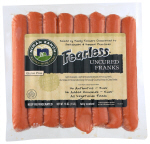16 oz
\$9.99

GF
6 oz
\$12.99

## Olli Gluten Free Napoli Salami

GF
6 oz
\$12.99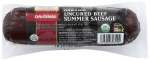## Organic Prairie Organic Beef Summer Sausage

CFLOG
12 oz
\$12.99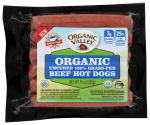CFGFLOG
10 oz
\$8.99

## Seward Co-op Andouille Pack Sausage

CFL
Each (price approx.)
\$15.98You are ordering units, but this item is sold by the pound. The price per pound is \$9.99 and the average weight for one item is 1.60 lbs., therefore, the approximate price for one item is \$15.98. Please order the number of items you would like to receive. Your final price will be calculated based on the total weight of the actual items fulfilled.

## Seward Co-op Andouille Sausage

CFL
Each (price approx.)
\$2.97You are ordering units, but this item is sold by the pound. The price per pound is \$8.99 and the average weight for one item is 0.33 lbs., therefore, the approximate price for one item is \$2.97. Please order the number of items you would like to receive. Your final price will be calculated based on the total weight of the actual items fulfilled.

## Seward Co-op Bierbrat

CFL
Each (price approx.)
\$3.24You are ordering units, but this item is sold by the pound. The price per pound is \$8.99 and the average weight for one item is 0.36 lbs., therefore, the approximate price for one item is \$3.24. Please order the number of items you would like to receive. Your final price will be calculated based on the total weight of the actual items fulfilled.

## Seward Co-op Bratwurst

CFL
Each (price approx.)
\$2.64You are ordering units, but this item is sold by the pound. The price per pound is \$7.99 and the average weight for one item is 0.33 lbs., therefore, the approximate price for one item is \$2.64. Please order the number of items you would like to receive. Your final price will be calculated based on the total weight of the actual items fulfilled.

## Seward Co-op Bratwurst Pack

CFL
Each (price approx.)
\$13.58You are ordering units, but this item is sold by the pound. The price per pound is \$8.49 and the average weight for one item is 1.60 lbs., therefore, the approximate price for one item is \$13.58. Please order the number of items you would like to receive. Your final price will be calculated based on the total weight of the actual items fulfilled.

## Seward Co-op Breakfast Sausage

CFL
Each (price approx.)
\$3.30You are ordering units, but this item is sold by the pound. The price per pound is \$9.99 and the average weight for one item is 0.33 lbs., therefore, the approximate price for one item is \$3.30. Please order the number of items you would like to receive. Your final price will be calculated based on the total weight of the actual items fulfilled.

## Seward Co-op Breakfast Sausage Chub

CFL
Each (price approx.)
\$2.97You are ordering units, but this item is sold by the pound. The price per pound is \$8.99 and the average weight for one item is 0.33 lbs., therefore, the approximate price for one item is \$2.97. Please order the number of items you would like to receive. Your final price will be calculated based on the total weight of the actual items fulfilled.

CFL
Per Pound
\$7.49
lb

CFL
Per Pound
\$6.99
lb

## Seward Co-op Calabrese Italian Sausage

CFL
Each (price approx.)
\$3.63You are ordering units, but this item is sold by the pound. The price per pound is \$10.99 and the average weight for one item is 0.33 lbs., therefore, the approximate price for one item is \$3.63. Please order the number of items you would like to receive. Your final price will be calculated based on the total weight of the actual items fulfilled.## Seward Co-op Chicken Hot Dog Pack

CFL
Each (price approx.)
\$9.99You are ordering units, but this item is sold by the pound. The price per pound is \$9.99 and the average weight for one item is 1.00 lbs., therefore, the approximate price for one item is \$9.99. Please order the number of items you would like to receive. Your final price will be calculated based on the total weight of the actual items fulfilled.

## Seward Co-op Chicken Italian Sausage

CFL
Each (price approx.)
\$2.47You are ordering units, but this item is sold by the pound. The price per pound is \$7.49 and the average weight for one item is 0.33 lbs., therefore, the approximate price for one item is \$2.47. Please order the number of items you would like to receive. Your final price will be calculated based on the total weight of the actual items fulfilled.

## Seward Co-op Chicken Provencal Sausage

CFL
Each (price approx.)
\$2.97You are ordering units, but this item is sold by the pound. The price per pound is \$8.99 and the average weight for one item is 0.33 lbs., therefore, the approximate price for one item is \$2.97. Please order the number of items you would like to receive. Your final price will be calculated based on the total weight of the actual items fulfilled.

## Seward Co-op Chorizo

CFL
Each (price approx.)
\$3.30You are ordering units, but this item is sold by the pound. The price per pound is \$9.99 and the average weight for one item is 0.33 lbs., therefore, the approximate price for one item is \$3.30. Please order the number of items you would like to receive. Your final price will be calculated based on the total weight of the actual items fulfilled.

## Seward Co-op Chorizo Chub

CFL
Each (price approx.)
\$8.99You are ordering units, but this item is sold by the pound. The price per pound is \$8.99 and the average weight for one item is 1.00 lbs., therefore, the approximate price for one item is \$8.99. Please order the number of items you would like to receive. Your final price will be calculated based on the total weight of the actual items fulfilled.

## Seward Co-op Currywurst

CFL
Each (price approx.)
\$2.97You are ordering units, but this item is sold by the pound. The price per pound is \$8.99 and the average weight for one item is 0.33 lbs., therefore, the approximate price for one item is \$2.97. Please order the number of items you would like to receive. Your final price will be calculated based on the total weight of the actual items fulfilled.

## Seward Co-op Fig and Shallot Sausage

CFL
Each (price approx.)
\$3.30You are ordering units, but this item is sold by the pound. The price per pound is \$9.99 and the average weight for one item is 0.33 lbs., therefore, the approximate price for one item is \$3.30. Please order the number of items you would like to receive. Your final price will be calculated based on the total weight of the actual items fulfilled.

## Seward Co-op Franklin Frank Pack

CFL
Each (price approx.)
\$9.99You are ordering units, but this item is sold by the pound. The price per pound is \$9.99 and the average weight for one item is 1.00 lbs., therefore, the approximate price for one item is \$9.99. Please order the number of items you would like to receive. Your final price will be calculated based on the total weight of the actual items fulfilled.

CFL
Per Pound
\$8.99
lb

## Seward Co-op Käsekrainer Sausage

CFL
Each (price approx.)
\$3.60You are ordering units, but this item is sold by the pound. The price per pound is \$9.99 and the average weight for one item is 0.36 lbs., therefore, the approximate price for one item is \$3.60. Please order the number of items you would like to receive. Your final price will be calculated based on the total weight of the actual items fulfilled.

## Seward Co-op Maple Breakfast Sausage

CFL
Each (price approx.)
\$2.97You are ordering units, but this item is sold by the pound. The price per pound is \$8.99 and the average weight for one item is 0.33 lbs., therefore, the approximate price for one item is \$2.97. Please order the number of items you would like to receive. Your final price will be calculated based on the total weight of the actual items fulfilled.

## Seward Co-op Mild Italian Sausage Chub

CFL
Each (price approx.)
\$6.99You are ordering units, but this item is sold by the pound. The price per pound is \$6.99 and the average weight for one item is 1.00 lbs., therefore, the approximate price for one item is \$6.99. Please order the number of items you would like to receive. Your final price will be calculated based on the total weight of the actual items fulfilled.

## Seward Co-op Polish Smoked Sausage

CFL
Each (price approx.)
\$2.97You are ordering units, but this item is sold by the pound. The price per pound is \$8.99 and the average weight for one item is 0.33 lbs., therefore, the approximate price for one item is \$2.97. Please order the number of items you would like to receive. Your final price will be calculated based on the total weight of the actual items fulfilled.

## Seward Co-op Spring Chicken Pack Sausage

CFL
Each (price approx.)
\$15.83You are ordering units, but this item is sold by the pound. The price per pound is \$10.99 and the average weight for one item is 1.44 lbs., therefore, the approximate price for one item is \$15.83. Please order the number of items you would like to receive. Your final price will be calculated based on the total weight of the actual items fulfilled.

## Seward Co-op Spring Chicken Sausage

CFL
Each (price approx.)
\$3.30You are ordering units, but this item is sold by the pound. The price per pound is \$9.99 and the average weight for one item is 0.33 lbs., therefore, the approximate price for one item is \$3.30. Please order the number of items you would like to receive. Your final price will be calculated based on the total weight of the actual items fulfilled.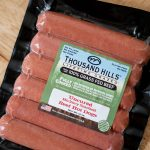CFGFL
10 oz
\$6.99

CFGFL
10 oz
\$8.99

CFL
2 oz
\$5.99